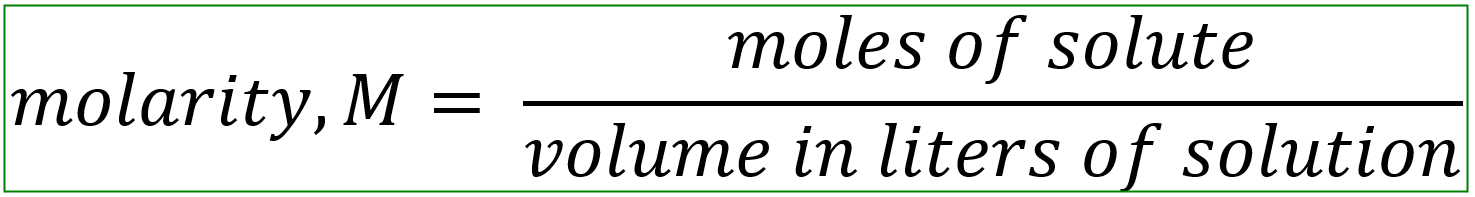# Problem: Calculate the molarity of each aqueous solution:(a) 0.82 g of ethanol (C2H5OH) in 10.5 mL of solution

###### FREE Expert Solution

Molarity is the moles of solute in 1 liter of the solution. It is calculated by:81% (160 ratings)###### Problem Details

Calculate the molarity of each aqueous solution:

(a) 0.82 g of ethanol (C2H5OH) in 10.5 mL of solution